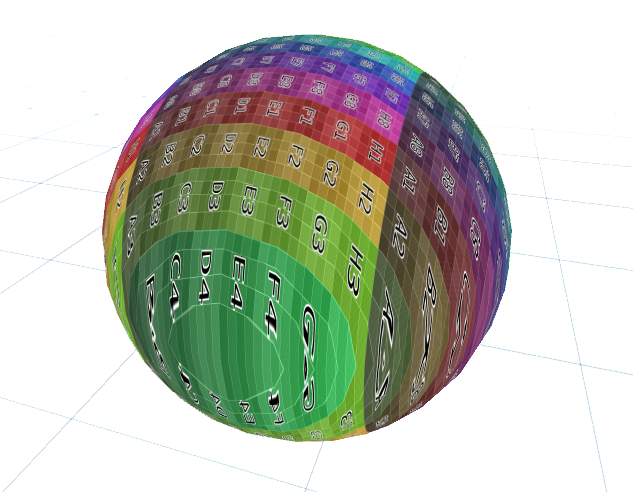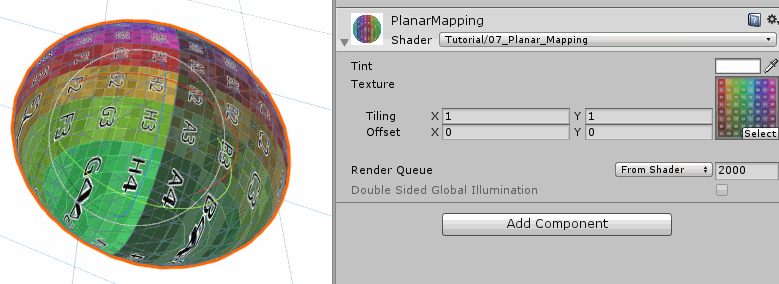## Summary

Sometimes you don’t have texture coordinates on your object, you want to make the Textures of multiple Objects align or you have a different reason to generate your own UV coordinates… In this tutorial we’ll start with the simplest way to generate your own coordinates, planar mapping.

This tutorial will build on the simple textured shader, but you can use the technique with any shader including surface shaders.## Basics

We start by removing the uv coordinates from the input struct as we’ll generate our own texture coordinates.

``````struct appdata{
float vertex : POSITION;
};
``````

Because UV coordinates can still be interpolated between the vertices like they were before, we calculate the new UVs in the vertex shader. As a start we can set the UV coordinates to the x and z values of the object coordinates. That’s enough to make the texture appear on our model and it looks like it’s pressed onto it from the top.

``````v2f vert(appdata v){
v2f o;
o.position = UnityObjectToClipPos(v.vertex);
o.uv = v.vertex.xz;
return o;
}
``````

This doesn’t take the texture scaling into consideration and we might not want the texture to rotate and move with the object as it does now.

To fix the texture scaling and offset, we just put the TRANSFORM_TEX macro around the uv coordinates.

``````v2f vert(appdata v){
v2f o;
o.position = UnityObjectToClipPos(v.vertex);
o.uv = TRANSFORM_TEX(v.vertex.xz, _MainTex);
return o;
}
``````## Texture Coordinates based on World Position

To take the object position and rotation out of the equation, we have to use the position of the vertex in the world (previously we used the position relative to the object center). To calculate the world position, we multiply the object to world matrix with it (I won’t go into matrix multiplication here). After we obtain the world position, we use that to set the uv coordinates.

``````v2f vert(appdata v){
v2f o;
//calculate the position in clip space to render the object
o.position = UnityObjectToClipPos(v.vertex);
//calculate world position of vertex
float4 worldPos = mul(unity_ObjectToWorld, v.vertex);
//change UVs based on tiling and offset of texture
o.uv = TRANSFORM_TEX(worldPos.xz, _MainTex);
return o;
}
``````As you see this technique has some disadvantages, mainly that it only works with tileable textures and the stretching on the sides, but that can be mitigated with some more advanced techniques like triplanar mapping which I’ll get into in a later tutorial.

``````Shader "Tutorial/008_Planar_Mapping"{
//show values to edit in inspector
Properties{
_Color ("Tint", Color) = (0, 0, 0, 1)
_MainTex ("Texture", 2D) = "white" {}
}

//the material is completely non-transparent and is rendered at the same time as the other opaque geometry
Tags{ "RenderType"="Opaque" "Queue"="Geometry"}

Pass{
CGPROGRAM

#include "UnityCG.cginc"

#pragma vertex vert
#pragma fragment frag

//texture and transforms of the texture
sampler2D _MainTex;
float4 _MainTex_ST;

fixed4 _Color;

struct appdata{
float4 vertex : POSITION;
};

struct v2f{
float4 position : SV_POSITION;
float2 uv : TEXCOORD0;
};

v2f vert(appdata v){
v2f o;
//calculate the position in clip space to render the object
o.position = UnityObjectToClipPos(v.vertex);
//calculate world position of vertex
float4 worldPos = mul(unity_ObjectToWorld, v.vertex);
//change UVs based on tiling and offset of texture
o.uv = TRANSFORM_TEX(worldPos.xz, _MainTex);
return o;
}

fixed4 frag(v2f i) : SV_TARGET{
fixed4 col = tex2D(_MainTex, i.uv);
//multiply texture color with tint color
col *= _Color;
return col;
}

ENDCG
}
}